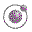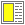Expressions, Types, and Functions - slide 35 : 46

Functions in Scheme

Functions are represented as lambda expressions in a source program

At run time, functions are represented as first class function objects

```> (define x 6)

> (lambda (x) (+ x 1))
#<procedure>

> (define inc (lambda (x) (+ x 1)))

> inc
#<procedure:inc>

> (if (even? x) inc fac)
#<procedure:inc>

> ((if (even? x) inc fac) 5)
6```